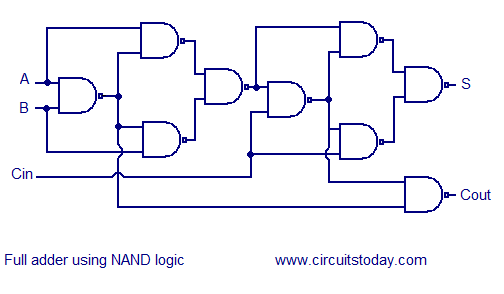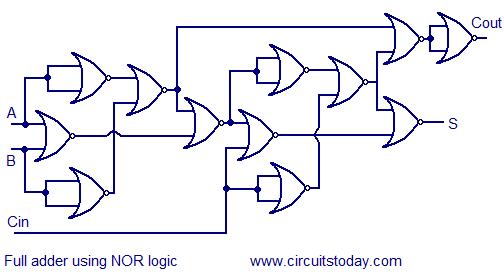Thursday, October 26, 2017

Circuit Schematic Full adder using NAND or NOR logic

Robometricschool. - This is one of the application of NAND and NOR logic to build full adder for you. Before you can build full adder with combining two half adder using logic circuit.

In here we will show you two circuit schematic of full adder using NAND and NOR logic look like shown in Figure 1 and Figure 2 below.

Circuit SchematicFigure 1. Circuit Schematic Full adder using NAND logic (Source: Circuitstoday.com)Figure 2. Circuit Schematic Full adder using NOR logic (Source: Circuitstoday.com)

Component Parts

• NAND Logic
• NOR Logic

Description

Accordingly Circuitstoday site that published this circuit describe that o understand the working of a ripple carry adder completely, you need to have a look at the full adder too. Full adder is a logic circuit that adds two input operand bits plus a Carry in bit and outputs a Carry out bit and a sum bit.. The Sum out  (Sout) of a full adder is the XOR of input operand bits A, B and the Carry in (Cin) bit.  Truth table and schematic of a 1 bit Full adder is shown below

There is a simple trick to find results of a full adder. Consider the second last row of the truth table, here the operands are 1, 1, 0 ie  (A, B, Cin). Add them together ie 1+1+0 = 10 . In binary system,  the number order is 0, 1, 10, 11……. and so the result of 1+1+0 is 10 just like we get 1+1+0 =2 in decimal system. 2 in the decimal system corresponds to 10 in the binary system. Swapping the result “10” will give S=0 and Cout = 1 and the second last row is justified.